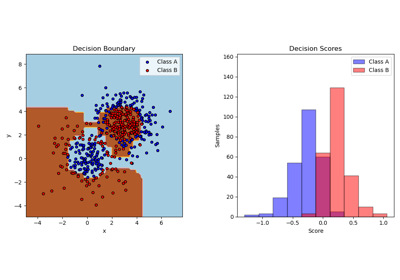# Ensemble methods¶

Examples concerning the sklearn.ensemble module.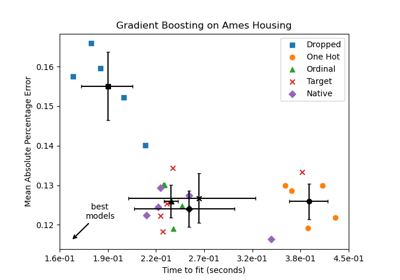Categorical Feature Support in Gradient Boosting

Categorical Feature Support in Gradient Boosting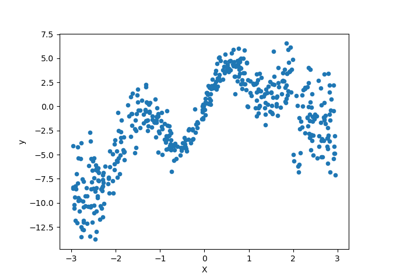Combine predictors using stacking

Combine predictors using stackingComparing Random Forests and Histogram Gradient Boosting models

Comparing Random Forests and Histogram Gradient Boosting models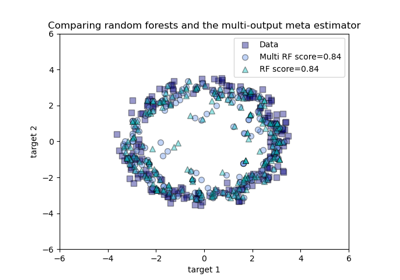Comparing random forests and the multi-output meta estimator

Comparing random forests and the multi-output meta estimator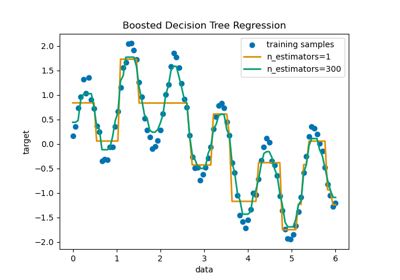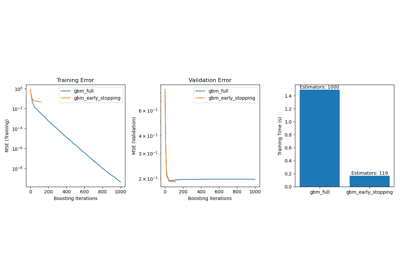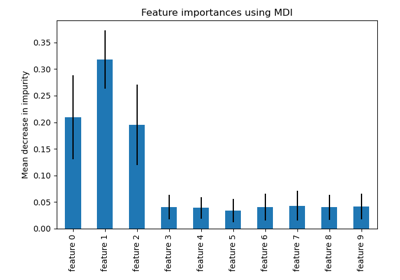Feature importances with a forest of trees

Feature importances with a forest of trees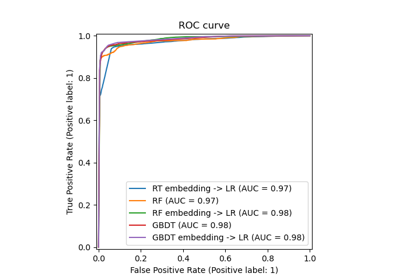Feature transformations with ensembles of trees

Feature transformations with ensembles of trees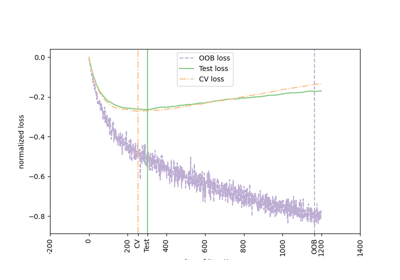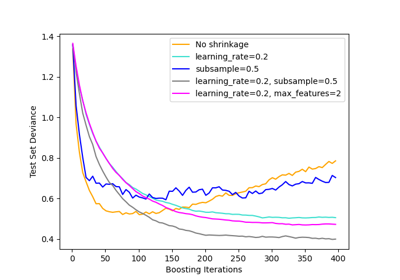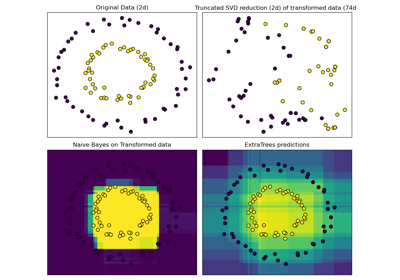Hashing feature transformation using Totally Random Trees

Hashing feature transformation using Totally Random Trees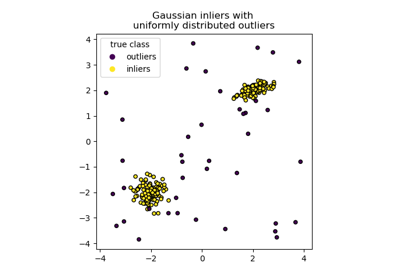IsolationForest example

IsolationForest example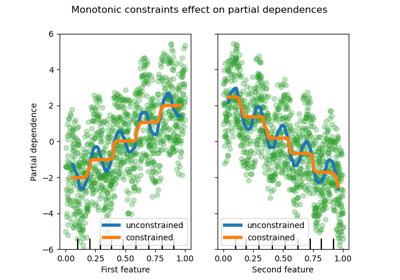Monotonic Constraints

Monotonic Constraints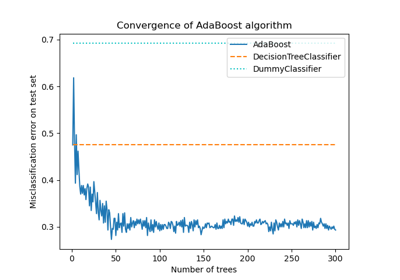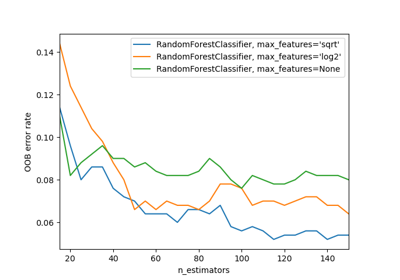OOB Errors for Random Forests

OOB Errors for Random ForestsPixel importances with a parallel forest of trees

Pixel importances with a parallel forest of treesPlot class probabilities calculated by the VotingClassifier

Plot class probabilities calculated by the VotingClassifier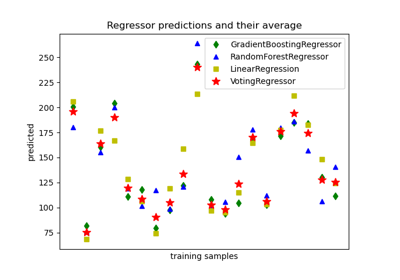Plot individual and voting regression predictions

Plot individual and voting regression predictions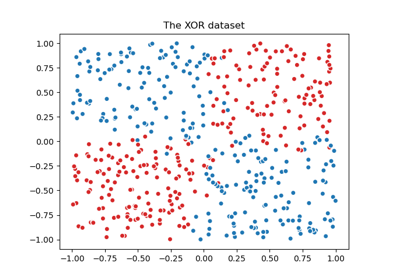Plot the decision boundaries of a VotingClassifier

Plot the decision boundaries of a VotingClassifier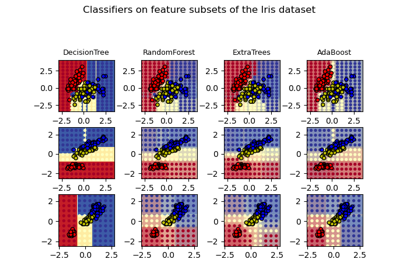Plot the decision surfaces of ensembles of trees on the iris dataset

Plot the decision surfaces of ensembles of trees on the iris dataset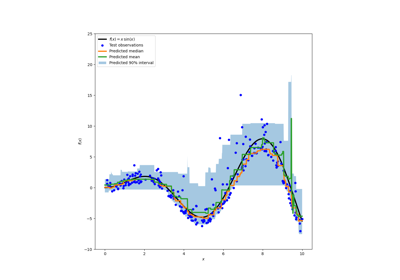Prediction Intervals for Gradient Boosting Regression

Prediction Intervals for Gradient Boosting Regression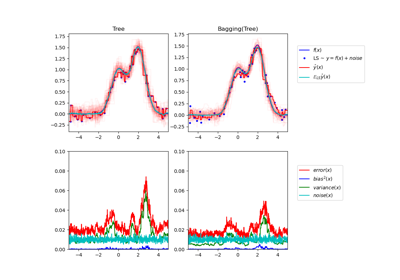Single estimator versus bagging: bias-variance decomposition

Single estimator versus bagging: bias-variance decomposition